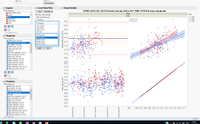Choose Language Hide Translation Bar
Highlighted

## Add check box on top of graph builder platform

Hi all.

I have a graph builder object with 3 column switchers and local data filter, I used "platform" to put it in a new window.I was wonder if there is an option to put "checkbox" on top of the graph builder in some free space? I know I can put it above/under/aside but I want it to stand out

Thanks

3 REPLIES 3
Highlighted

## Re: Add check box on top of graph builder platform

It looks like you have your own display boxes about the Graph Builder with its data filters. Can't you extend that part of your display tree to add a Check Box? What is the code you use for the portion of the window above Graph Builder?

Learn it once, use it forever!
Highlighted

## Re: Add check box on top of graph builder platform

The filters are not in the display box, they are just how JMP sort them. Here is the code:

``````nw = new window(

Platform(
dt,
gb = Graph Builder(
Size( 865, 775 ),
Show Control Panel( 0 ),
Fit to Window( "Off" ),
Variables(
X( :TIME_GTREC_135153 ),
X( :TREC_GH_135147 ),
Y( :GTREC_GH_135153 ),
Y( :TREC_GH_135147 ),
Group X( :Prod ),
Overlay( :FAB, :DEPOP_ONT_SITE )
),
Elements( Position( 1, 1 ), Points( X, Y, Legend( 36 ) ), Smoother( X, Y, Legend( 48 ) ) ),
Elements( Position( 1, 2 ), Points( X, Y, Legend( 38 ) ), Smoother( X, Y, Legend( 49 ) ) ),
Elements( Position( 2, 1 ), Points( X, Y, Legend( 40 ) ), Line Of Fit( X, Y, Legend( 24 ), R²( 1 ) ) ),
Elements( Position( 2, 2 ), Points( X, Y, Legend( 42 ) ), Smoother( X, Y, Legend( 51 ) ) ),
);

),

ldf = gb << Local Data Filter(
columns( :FAB, :Prod ), //, :TIME_GTREC_135153 ),
Display( :FAB, Size( 160, 60 ), List Display ),
Display( :Prod, Size( 160, 60 ), List Display ),
Where( :prod = "8PEQ" ),
//Display( :TIME_GTREC_135153),
Order By Count( :Prod )
)

);

cso1 = gb << Column Switcher( :FAB, legend_cls );
cso2 = gb << Column Switcher( :GTREC_GH_135153, num_cls );
cso3 = gb << Column Switcher( :TREC_GH_135147, num_cls );
cso4 = gb << Column Switcher( :TIME_GTREC_135153, time_cls );
cso5 = gb << Column Switcher( :Prod, {:Prod, :Const} );
cso1 << Set Size( 200 );
cso1 << Set NLines( 6 );
cso2 << Set Size( 200 );
cso2 << Set NLines( 15 );
cso3 << Set Size( 200 );
cso3 << Set NLines( 15 );
cso4 << Set Size( 200 );
cso4 << Set NLines( 10 );
cso5 << Set Size( 200 );
cso5 << Set NLines( 2 );
);``````
Highlighted

## Re: Add check box on top of graph builder platform

If you wanted the checkbox close to the graphic display, I'd probably use the code below, assuming gb is the graph builder object and rgb is its report.

`` ( (rgb[PictureBox(1)] << Parent ) << Child ) << Sib Append (mychb);``

Here is the picture. Script attached.Article Labels

There are no labels assigned to this post.